# ⚙ Usage

## 🚀 Basics

### 🌐 Initialization of Maps objects

EOmaps is all about Maps objects.
To start creating a new map (in this case a plot in epsg=4326, e.g. lon/lat), simply use:
from eomaps import Maps
m = Maps(crs=4326, layer="first layer", figsize=(10, 5))

• crs represents the projection used for plotting

• layer represents the name of the layer associated with the Maps-object (see below)

• all additional keyword arguments are forwarded to the creation of the matplotlib-figure (e.g.: figsize, frameon, edgecolor etc).

Possible ways for specifying the crs for plotting are:

• If you provide an integer, it is identified as an epsg-code (e.g. 4326, 3035, etc.).

• All other CRS usable for plotting are accessible via Maps.CRS, e.g.: crs=Maps.CRS.Orthographic() or crs=Maps.CRS.Equi7Grid_projection("EU"). (Maps.CRS is just an accessor for cartopy.crs)

### ▤ Layers

Each Maps object represents an individual plot-layer of the map.
Once you have created your first Maps object, you can create additional layers on the same map by using:
m = Maps()                           # same as m = Maps(layer=0)
m2 = m.new_layer()                   # "m2" is just another Maps-object on the same layer as "m"!

m_ocean = m.new_layer(layer="ocean") # create a new layer named "ocean"
m_ocean.add_feature.preset.ocean()   # features on this layer will only be visible if the "ocean" layer is visible!
m.show_layer("ocean")                # show the "ocean" layer

m.all.add_feature.preset.coastline() # to add a feature to all layers, simply add it to the all layer with m.all...

m.util.layer_selector()              # get a utility widget to simplify switching between existing layers

• m2, m_ocean and m.all are just ordinary Maps objects that share the figure and plot-axes with m

• If you don’t provide an explicit layer name, the new Maps-object will use the same layer as its parent! (you can have multiple Maps objects on the same layer!)

Map-features and colorbars are layer-sensitive!

Features, datasets, colormaps etc. added to a Maps object are only visible if the associated layer is visible! To manually switch between layers, use m.show_layer("the layer name"), call m.show() or have a look at the 🦜 Utility widgets.

The “all” layer

There is one layer-name that has a special meaning… the "all" layer.
Any callbacks, features or plots added to this layer will be executed on all other layers as well!

You can add features and callbacks to the all layer via: - using the shortcut m.all. ... - creating a dedicated Maps object via m_all = Maps(layer="all") or m_all = m.new_layer("all") - using the “layer” kwarg of functions e.g. m.plot_map(layer="all")

 Maps The base-class for generating plots with EOmaps. Maps.new_layer Create a new Maps-object that shares the same plot-axes. Maps.all Get a Maps-object on the "all" layer. Maps.show_layer Display the selected layer on the map. Maps.copy Create a (deep)copy of the Maps object that shares selected specifications.

### 🔵 Setting the data and plot-shape

To assign a dataset to a Maps object, use m.set_data(...). The shapes that are used to represent the data-points are then assigned via m.set_shape.

What’s used by default?

By default, the plot-shape is assigned based on the associated dataset.

• For datasets with less than 500 000 pixels, m.set_shape.ellipses() is used.

• For larger 2D datasets m.set_shape.shade_raster() is used
… and m.set_shape.shade_points() is used for the rest.
m = Maps()                                # create a Maps-object
m.set_data(data1, x, y, crs, ...)         # assign some data to the Maps-object
n=100)           # use 100 intermediate points to represent the shape

m.plot_map()                              # plot the data

m2 = m.new_layer()                        # create a new Maps-object on the same layer
m2.set_data(data, x, y, crs, ...)         # assign another dataset to the new Maps object
m2.set_shape.rectangles(radius=1,         # represent the datapoints as 1x1 degree rectangles
m2.plot_map()                             # plot the data

m3 = m.new_layer("data")                  # create a new layer named "data"
...                                       # ...

 Maps.set_data Set the properties of the dataset you want to plot. Maps.set_shape Set the plot-shape to represent the data-points.

Possible shapes that work nicely for datasets with up to 1M data-points:

 geod_circles Draw geodesic circles with a radius defined in meters. ellipses Draw projected ellipses with dimensions defined in units of a given crs. rectangles Draw projected rectangles with fixed dimensions (and possibly curved edges) voronoi_diagram Draw a Voronoi-Diagram of the data. delaunay_triangulation Draw a Delaunay-Triangulation of the data. raster Draw 2D datasets as rectangles (only 2D datasets, but possibly large ones)

While raster still works nicely for large datasets , for extremely large datasets (several million datapoints), it is recommended to use “shading” instead of representing each data-point with a projected polygon.

Possible shapes that can be used to quickly generate a plot for millions of datapoints are:

 shade_points Shade the data as infinitesimal points (>> usable for very large datasets!). shade_raster Shade the data as a rectangular raster (>> usable for very large datasets!).

If shading is used, a dynamic averaging of the data based on the screen-resolution and the currently visible plot-extent is performed (resampling based on the mean-value is used by default).

Note

The “shade”-shapes require the additional datashader dependency! You can install it via: conda install -c conda-forge datashader

To get an overview of the existing shapes and their main use-cases, here’s a simple decision-tree:### 🗺 Plot the map and save it

If you want to plot a map based on a dataset, first set the data and then call m.plot_map().

Any additional keyword-arguments passed to m.plot_map() are forwarded to the actual plot-command for the selected shape.

Some useful arguments that are supported by most shapes (except “shade”-shapes) are:

• “fc” or “facecolor” : the face-color of the shapes

• “ec” or “edgecolor” : the edge-color of the shapes

• “lw” or “linewidth” : the linewidth of the shapes

• “alpha” : the alpha-transparency

m = Maps()

m2 = m.new_layer("a data layer")
m2.set_data(...)
...
m2.plot_map(cmap="viridis", ec="g", lw=2, alpha=0.5)


To adjust the margins of the subplots, use m.subplots_adjust, e.g.:

m = Maps()

 Maps.subplots_adjust Update the subplot parameters of the grid.

You can then continue to add 🌈 Colorbars (with a histogram), 🏕 Annotations and Markers, 📏 Scalebars, 🧭 Compass (or North Arrow), 🛰 WebMap layers or 🌵 GeoDataFrames and NaturalEarth features to the map, or you can start to add 🦜 Utility widgets and 🛸 Callbacks - make the map interactive!.

Once the map is ready, a snapshot of the map can be saved at any time by using:

m.savefig( "snapshot1.png", dpi=300, ... )

 Maps.plot_map Actually generate the map-plot based on the data provided as m.data and the specifications defined in "data_specs" and "classify_specs". Maps.savefig Save the current figure.

### 🌍 Customizing the plot

All arguments to customize the appearance of a dataset are passed to m.plot_map(...).

In general, the colors assigned to the shapes are specified by selecting a colormap (cmap) and (optionally) setting appropriate limits via vmin and vmax.

• cmap can be specified as (see matplotlib-docs for more details):

• a name of a pre-defined matplotlib colormap (e.g. "viridis", "RdYlBu" etc.)

• or a general matplotlib colormap object

• vmin and vmax set the range of data-values that are mapped

• (Any values outside this range will get the colormaps over and under colors assigned.)

m = Maps()
m.set_data(...)
m.plot_map(cmap="viridis", vmin=0, vmax=1)


Colors can also be set manually by providing one of the following arguments to m.plot_map(...):

• to set both facecolor AND edgecolor use color=...

• to set the facecolor use fc=... or facecolor=...

• to set the edgecolor use ec=... or edgecolor=...

Note

• Manual color specifications do NOT work with the shade shapes!

• Providing manual colors will override the colors assigned by the cmap!

• The colorbar will NOT represent manually defined colors!

#### Uniform colors

To apply a uniform color to all datapoints, you can use matpltolib’s color-names or pass an RGB or RGBA tuple.

m.plot_map(fc="r")
m.plot_map(fc="orange")
m.plot_map(fc=(1, 0, 0.5))
m.plot_map(fc=(1, 0, 0.5, .25))
# for grayscale use a string of a number between 0 and 1
m.plot_map(fc="0.3")


#### Explicit colors

To explicitly color each datapoint with a pre-defined color, simply provide a list or array of the aforementioned types.

m.plot_map(fc=["r", "g", "orange"])
# for grayscale use a string of a number between 0 and 1
m.plot_map(fc=[".1", ".2", "0.3"])
# or use RGB / RGBA tuples
m.plot_map(fc=[(1, 0, 0.5), (.3, .4, .5), (1, 1, 0)])
m.plot_map(fc=[(1, 0, 0.5, .25), (1, 0, 0.5, .75), (.1, .2, 0.5, .5)])


#### RGB composites

To create an RGB or RGBA composite from 3 (or 4) datasets, pass the datasets as tuple:

• the datasets must have the same size as the coordinate arrays!

• the datasets must be scaled between 0 and 1

# if you pass a tuple of 3 or 4 arrays, they will be used to set the
# RGB (or RGBA) colors of the shapes
m.plot_map(fc=(<R-array>, <G-array>, <B-array>))
m.plot_map(fc=(<R-array>, <G-array>, <B-array>, <A-array>))

# you can fix individual color channels by passing a list with 1 element
m.plot_map(fc=(<R-array>, [0.12345], <B-array>, <A-array>))


### 📊 Data classification

EOmaps provides an interface for mapclassify to classify datasets prior to plotting via m.set_classify_specs. Available classifiers that can be used are accessible via Maps.CLASSIFIERS:

m = Maps()
m.set_data(...)
m.set_shape.ellipses(...)

m.set_classify_specs(Maps.CLASSFIERS.Quantiles, k=5)
m.classify_specs.k = 10 # alternative way for setting classify-specs

 Maps.set_classify_specs Set classification specifications for the data.

Currently available classification-schemes are (see mapclassify for details):

• BoxPlot (hinge)

• EqualInterval (k)

• FisherJenks (k)

• FisherJenksSampled (k, pct, truncate)

• JenksCaspall (k)

• JenksCaspallForced (k)

• JenksCaspallSampled (k, pct)

• MaxP (k, initial)

• MaximumBreaks (k, mindiff)

• NaturalBreaks (k, initial)

• Quantiles (k)

• Percentiles (pct)

• StdMean (multiples)

• UserDefined (bins)

### 𝄜 Multiple maps in one figure

MapsGrid objects can be used to create (and manage) multiple maps in one figure.

A MapsGrid creates a grid of Maps objects (and/or ordinary matpltolib axes), and provides convenience-functions to perform actions on all maps of the figure.

from eomaps import MapsGrid
mg = MapsGrid(r=2, c=2, crs=..., layer=..., ... )
# you can then access the individual Maps-objects via:
mg.m_0_0
mg.m_0_1
mg.m_1_0
mg.m_1_1

m2 = mg.m_0_0.new_layer("newlayer")
...

# there are many convenience-functions to perform actions on all Maps-objects:
...

# to perform more complex actions on all Maps-objects, simply loop over the MapsGrid object
for m in mg:
...

# set the margins of the plot-grid
mg.subplots_adjust(left=0.1, right=0.9, bottom=0.05, top=0.95, hspace=0.1, wspace=0.05)


#### Custom grids and mixed axes

Fully customized grid-definitions can be specified by providing m_inits and/or ax_inits dictionaries of the following structure:

• The keys of the dictionary are used to identify the objects

• The values of the dictionary are used to identify the position of the associated axes

• The position can be either an integer N, a tuple of integers or slices (row, col)

• Axes that span over multiple rows or columns, can be specified via slice(start, stop)

dict(
name1 = N  # position the axis at the Nth grid cell (counting firs)
name2 = (row, col), # position the axis at the (row, col) grid-cell
name3 = (row, slice(col_start, col_end)) # span the axis over multiple columns
name4 = (slice(row_start, row_end), col) # span the axis over multiple rows
)

• m_inits is used to initialize Maps objects

• ax_inits is used to initialize ordinary matplotlib axes

The individual Maps-objects and matpltolib-Axes are then accessible via:

mg = MapsGrid(2, 3,
m_inits=dict(left=(0, 0), right=(0, 2)),
ax_inits=dict(someplot=(1, slice(0, 3)))
)
mg.m_left   # the Maps object with the name "left"
mg.m_right   # the Maps object with the name "right"

mg.ax_someplot   # the ordinary matplotlib-axis with the name "someplot"


❗ NOTE: if m_inits and/or ax_inits are provided, ONLY the explicitly defined objects are initialized!

• The initialization of the axes is based on matplotlib’s GridSpec functionality. All additional keyword-arguments (width_ratios, height_ratios, etc.) are passed to the initialization of the GridSpec object.

• To specify unique crs for each Maps object, provide a dictionary of crs specifications.

from eomaps import MapsGrid

# initialize a grid with 2 Maps objects and 1 ordinary matplotlib axes
mgrid = MapsGrid(2, 2,
m_inits=dict(top_row=(0, slice(0, 2)),
bottom_left=(1, 0)),
crs=dict(top_row=4326,
bottom_left=3857),
ax_inits=dict(bottom_right=(1, 1)),
width_ratios=(1, 2),
height_ratios=(2, 1))

mgrid.m_top_row # a map extending over the entire top-row of the grid (in epsg=4326)
mgrid.m_bottom_left # a map in the bottom left corner of the grid (in epsg=3857)

mgrid.ax_bottom_right # an ordinary matplotlib axes in the bottom right corner of the grid

 MapsGrid Initialize a grid of Maps objects MapsGrid.join_limits Join axis limits between all Maps objects of the grid (only possible if all maps share the same crs!) MapsGrid.share_click_events Share click events between all Maps objects of the grid MapsGrid.share_pick_events Share pick events between all Maps objects of the grid MapsGrid.set_data_specs This will execute the corresponding action on ALL Maps objects of the MapsGrid! MapsGrid.set_classify_specs This will execute the corresponding action on ALL Maps objects of the MapsGrid! MapsGrid.add_wms A collection of open-access WebMap services that can be added to the maps MapsGrid.add_feature Interface to the feature-layers provided by NaturalEarth MapsGrid.add_annotation This will execute the corresponding action on ALL Maps objects of the MapsGrid! MapsGrid.add_marker This will execute the corresponding action on ALL Maps objects of the MapsGrid! MapsGrid.add_gdf This will execute the corresponding action on ALL Maps objects of the MapsGrid!

## 🛸 Callbacks - make the map interactive!

Callbacks are used to execute functions when you click on the map.

They can be attached to a map via:

m = Maps()
...
m.cb.< METHOD >.attach.< CALLBACK >( **kwargs )


< METHOD > defines the way how callbacks are executed.

 click Callbacks that are executed if you click anywhere on the Map. pick Callbacks that select the nearest datapoint if you click on the map. keypress Callbacks that are executed if you press a key on the keyboard. dynamic Callbacks that are triggered by events in the map (e.g.

< CALLBACK > indicates the action you want to assign o the event. There are many pre-defined callbacks, but it is also possible to define custom functions and attach them to the map.

Note

Callbacks are only executed if the layer of the associated Maps object is actually visible! (This assures that pick-callbacks always refer to the visible dataset.)

To define callbacks that are executed independent of the visible layer, attach it to the "all" layer using something like m.all.cb.click.attach.annotate().

 from eomaps import Maps import numpy as np x, y = np.mgrid[-45:45, 20:60] m = Maps(Maps.CRS.Orthographic()) m.all.add_feature.preset.coastline() m.set_data(data=x+y**2, x=x, y=y, crs=4326) m.plot_map(pick_distance=10) m2 = m.new_layer(copy_data_specs=True, layer="second_layer") m2.plot_map(cmap="tab10") # get an annotation if you RIGHT-click anywhere on the map m.cb.click.attach.annotate(xytext=(-60, -60), bbox=dict(boxstyle="round", fc="r")) # pick the nearest datapoint if you click on the MIDDLE mouse button m.cb.pick.attach.annotate(button=2) m.cb.pick.attach.mark(buffer=1, permanent=False, fc="none", ec="r", button=2) m.cb.pick.attach.mark(buffer=4, permanent=False, fc="none", ec="r", button=2) # peek at the second layer if you LEFT-click on the map m.cb.click.attach.peek_layer("second_layer", how=.25, button=3)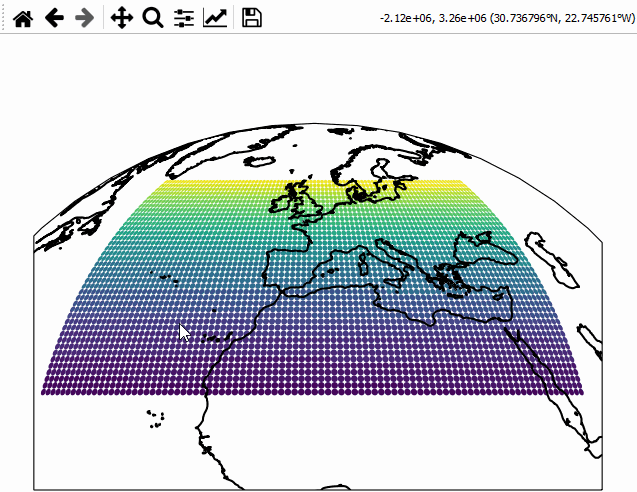### Pre-defined click & pick callbacks

Callbacks that can be used with both m.cb.click and m.cb.pick:

 peek_layer Swipe between data- or WebMap layers or peek a layers through a rectangle. annotate Add a basic text-annotation to the plot at the position where the map was clicked. clear_annotations Remove all temporary and permanent annotations from the plot mark Draw markers at the location where the map was clicked. clear_markers Remove all temporary and permanent annotations from the plot. get_values Successively collect return-values in a dict accessible via m.cb.[click/pick].get.picked_vals. print_to_console Print details on the clicked pixel to the console

Callbacks that can be used only with m.cb.pick:

 load Load objects from a given database using the ID of the picked pixel. highlight_geometry Temporarily highlite the picked geometry.

### Pre-defined keypress callbacks

Callbacks that can be used with m.cb.keypress

 switch_layer Change the default layer of the map.

### Pre-defined dynamic callbacks

Callbacks that can be used with m.cb.dynamic

 indicate_extent Indicate the plot-extent of another maps object.

### Custom callbacks

Custom callback functions can be attached to the map via:

def some_callback(asdf, **kwargs):
print("hello world")
print("the value of 'asdf' is", asdf)
print("the position of the clicked pixel in plot-coordinates", kwargs["pos"])
print("the dataset-index of the nearest datapoint", kwargs["ID"])
print("data-value of the nearest datapoint", kwargs["val"])
...

# attaching custom callbacks works completely similar for "click", "pick" and "keypress"!
m = Maps()
...
m.cb.pick.attach(some_callback, double_click=False, button=1, asdf=1)
m.cb.click.attach(some_callback, double_click=False, button=2, asdf=1)
m.cb.keypress.attach(some_callback, key="x", asdf=1)

• ❗ for click callbacks the kwargs ID and val are set to None!

• ❗ for keypress callbacks the kwargs ID and val and pos are set to None!

### Picking a dataset without plotting it first

It is possible to attach pick callbacks to a Maps object without plotting the data first by using m.make_dataset_pickable().

m = Maps()
m.set_data(... the dataset ...)
m.make_dataset_pickable()
# now it's possible to attach pick-callbacks even though the data is still "invisible"
m.cb.pick.attach.annotate()


Note

Using m.make_dataset_pickable() is ONLY necessary if you want to use pick callbacks without actually plotting the data! Otherwise a call to m.plot_map() is sufficient!

 make_dataset_pickable Make the associated dataset pickable without plotting it first.

## 🛰 WebMap layers

WebMap services (TS/WMS/WMTS) can be attached to the map via:

m.add_wms.attach.< SERVICE > ... .add_layer.< LAYER >(...)


< SERVICE > hereby specifies the pre-defined WebMap service you want to add, and < LAYER > indicates the actual layer-name.

m = Maps(Maps.CRS.GOOGLE_MERCATOR) # (the native crs of the service)

 Maps.add_wms A collection of open-access WebMap services that can be added to the maps

Note

It is highly recommended (and sometimes even required) to use the native crs of the WebMap service in order to avoid re-projecting the images (which degrades image quality and sometimes takes quite a lot of time to finish…)

• most services come either in epsg=4326 or in Maps.CRS.GOOGLE_MERCATOR projection

 from eomaps import Maps, MapsGrid mg = MapsGrid(crs=Maps.CRS.GOOGLE_MERCATOR) mg.join_limits() mg.m_0_0.add_wms.OpenStreetMap.add_layer.default() mg.m_0_1.add_wms.OpenStreetMap.add_layer.stamen_toner() mg.m_1_1.add_wms.S1GBM.add_layer.vv() # ... for more advanced layer = mg.m_1_0.add_wms.ISRIC_SoilGrids.nitrogen.add_layer.nitrogen_0_5cm_mean layer.set_extent_to_bbox() # set the extent according to the boundingBox layer.info # the "info" property provides useful informations on the layer layer() # call the layer to add it to the map layer.add_legend() # if a legend is provided, you can add it to the map!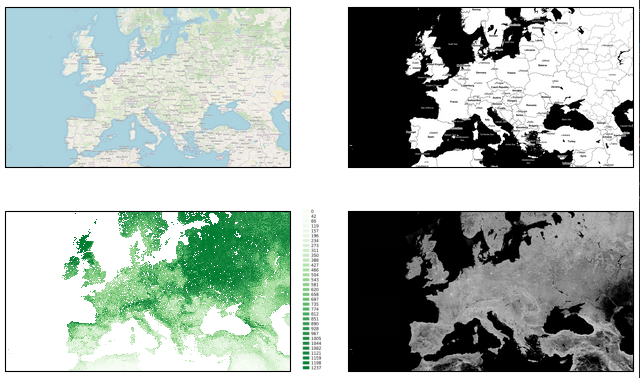### Pre-defined (global) WebMap services:

 OpenStreetMap (global) OpenStreetMap WebMap layers https://wiki.openstreetmap.org/wiki/WMS ESA_WorldCover ESA Worldwide land cover mapping https://esa-worldcover.org/en NASA_GIBS NASA Global Imagery Browse Services (GIBS) https://wiki.earthdata.nasa.gov/display/GIBS/ ISRIC_SoilGrids Interface to the ISRIC SoilGrids database https://www.isric.org/explore/soilgrids/faq-soilgrids EEA_DiscoMap European Environment Agency Discomap services https://discomap.eea.europa.eu/Index/ ESRI_ArcGIS Interface to the ERSI ArcGIS REST Services Directory http://services.arcgisonline.com/arcgis/rest/services S1GBM Sentinel-1 Global Backscatter Model https://researchdata.tuwien.ac.at/records/n2d1v-gqb91 S2_cloudless Global cloudless Sentinel-2 maps, crafted by EOX. GEBCO Global ocean & land terrain models https://www.gebco.net/

Services specific for Austria (Europa)

 Austria.AT_basemap Basemap for Austria https://basemap.at/ Austria.Wien_basemap Basemaps for the city of Vienna (Austria) https://www.wien.gv.at

Note

Services might be nested directory structures! The actual layer is always added via the add_layer directive.

m.add_wms.<...>. ... .<...>.add_layer.<LAYER NAME>()

Some of the services dynamically fetch the structure via HTML-requests. Therefore it can take a short moment before autocompletion is capable of showing you the available options! A list of available layers from a sub-folder can be fetched via:

m.add_wms.<...>. ... .<LAYER NAME>.layers

## 🌵 GeoDataFrames and NaturalEarth features

### 💠 GeoDataFrames

A geopandas.GeoDataFrame can be added to the map via m.add_gdf().

 Maps.add_gdf Plot a geopandas.GeoDataFrame on the map.
import geopandas as gpd

gdf = gpd.GeoDataFrame(geometries=[...], crs=...)

m = Maps()


It is possible to make the shapes of a GeoDataFrame pickable (e.g. usable with m.cb.pick callbacks) by providing a picker_name (and optionally specifying a pick_method).

Once the picker_name is specified, pick-callbacks can be attached via:

• m.cb.pick[<PICKER NAME>].attach.< CALLBACK >()

For example, to highlight the clicked country, you could use:
 from eomaps import Maps m = Maps() # get the GeoDataFrame for a given NaturalEarth feature gdf = m.add_feature.cultural_110m.admin_0_countries.get_gdf() # pick the shapes of the GeoDataFrame based on a "contains" query m.add_gdf(gdf, picker_name="countries", pick_method="contains") # temporarily highlight the picked geometry m.cb.pick["countries"].attach.highlight_geometry(fc="r", ec="g", lw=2)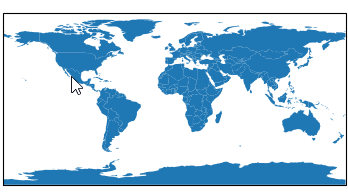### 🌴 NaturalEarth features

Feature-layers provided by NaturalEarth can be directly added to the plot via m.add_feature.

 Maps.add_feature Interface to the feature-layers provided by NaturalEarth

The general call-signature is:

m = Maps()
# just call the feature to add it to the map

# if you only want to get the associated GeoDataFrame, you can use
gdf = m.add_feature.< CATEGORY >.< FEATURE >.get_gdf()


Where < CATEGORY > specifies the resolution and general category of the feature, e.g.:

• cultural_10m, cultural_50m, cultural_110m: cultural features (e.g. countries, states etc.)

• physical_10m, physical_50m, physical_110m: physical features (e.g. coastline, land, ocean etc.)

The most commonly used features are available under the preset category:

 coastline Add a coastline to the map. ocean Add ocean-coloring to the map. land Add a land-coloring to the map. countries Add country-boundaries to the map.
 from eomaps import Maps m = Maps() m.add_feature.preset.coastline() m.add_feature.preset.ocean() m.add_feature.preset.land() m.add_feature.preset.countries() m.add_feature.physical_110m.lakes(ec="b") m.add_feature.cultural_110m.admin_0_pacific_groupings(ec="m", lw=2) # (only if geopandas is installed) places = m.add_feature.cultural_110m.populated_places.get_gdf() m.add_gdf(places, markersize=places.NATSCALE/10, fc="r")Note

If geopandas is installed, GeoDataFrames are used to visualize the features, and all aforementioned functionalities of m.add_gdf can also directly be used with m.add_feature!

## 🏕 Annotations and Markers

### 🔴 Markers

Static markers can be added to the map via m.add_marker().

• If a dataset has been plotted, you can mark any datapoint via its ID, e.g. ID=...

• To add a marker at an arbitrary position, use xy=(...)

• By default, the coordinates are assumed to be provided in the plot-crs

• You can specify arbitrary coordinates via xy_crs=...

• The radius is defined via radius=...

• By default, the radius is assumed to be provided in the plot-crs

• You can specify the radius in an arbitrary crs via radius_crs=...

• The marker-shape is set via shape=...

• Possible arguments are "ellipses", "rectangles", "geod_circles"

• Additional keyword-arguments are passed to the matplotlib collections used to draw the shapes (e.g. “facecolor”, “edgecolor”, “linewidth”, “alpha”, etc.)

• Multiple markers can be added in one go by using lists for xy, radius, etc.

🛸 For dynamic markers checkout m.cb.click.attach.mark() or m.cb.pick.attach.mark()

 add_marker add a marker to the plot
 from eomaps import Maps m = Maps(crs=4326) m.add_feature.preset.coastline() # ----- SINGLE MARKERS # by default, MARKER DIMENSIONS are defined in units of the plot-crs! m.add_marker(xy=(0, 0), radius=20, shape="rectangles", fc="y", ec="r", ls=":", lw=2) m.add_marker(xy=(0, 0), radius=10, shape="ellipses", fc="darkorange", ec="r", ls=":", lw=2) # MARKER DIMENSIONS can be specified in any CRS! m.add_marker(xy=(12000000, 0), xy_crs=3857, radius=5000000, radius_crs=3857, fc=(.5, .5, 0, .4), ec="r", lw=3, n=100) # GEODETIC CIRCLES with radius defined in meters m.add_marker(xy=(-135, 35), radius=3000000, shape="geod_circles", fc="none", ec="r", hatch="///", lw=2, n=100) # ----- MULTIPLE MARKERS x = [-80, -40, 40, 80] # x-coordinates of the markers fc = ["r", "g", "b", "c"] # the colors of the markers # N markers with the same radius m.add_marker(xy=(x, [-60]*4), radius=10, fc=fc) # N markers with different radius and properties m.add_marker(xy=(x, *4), radius=[15, 10, 5, 2], fc=fc, ec=["none", "r", "g", "b"], alpha=[1, .5, 1, .5]) # N markers with different widths and heights radius = ([15, 10, 5, 15], [5, 15, 15, 2]) m.add_marker(xy=(x, *4), radius=radius, fc=fc)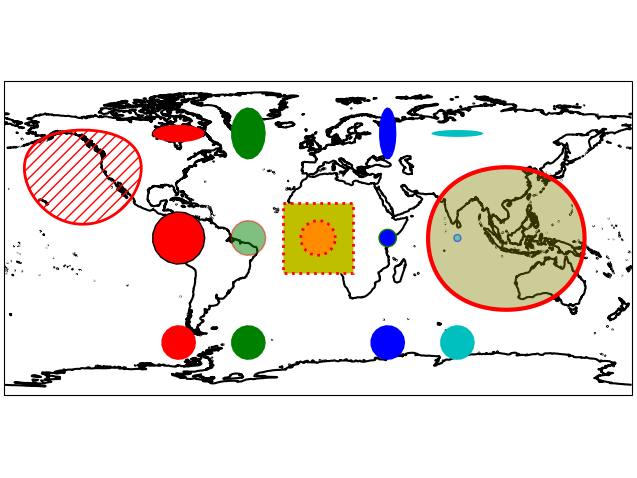### 📑 Annotations

Static annotations can be added to the map via m.add_annotation().

• The location is defined completely similar to m.add_marker() above.

• You can annotate a datapoint via its ID, or arbitrary coordinates in any crs.

• Additional arguments are passed to matplotlibs plt.annotate and plt.text

• This gives a lot of flexibility to style the annotations!

🛸 For dynamic annotations checkout m.cb.click.attach.annotate() or m.cb.pick.attach.annotate()

 from eomaps import Maps import numpy as np x, y = np.mgrid[-45:45, 20:60] m = Maps(crs=4326) m.set_data(x+y, x, y) m.add_feature.preset.coastline(ec="k", lw=.75) m.plot_map() # annotate any point in the dataset via the data-index m.add_annotation(ID=345) # annotate an arbitrary position (in the plot-crs) m.add_annotation( xy=(20,25), text="A formula:\n $z=\sqrt{x^2+y^2}$", fontweight="bold", bbox=dict(fc=".6", ec="none", pad=2)) # annotate coordinates defined in arbitrary crs m.add_annotation( xy=(2873921, 6527868), xy_crs=3857, xytext=(5,5), text="A location defined \nin epsg 3857", fontsize=8, rotation=-45, bbox=dict(fc="skyblue", ec="k", ls="--", pad=2)) # functions can be used for more complex text def text(m, ID, val, pos, ind): return f"lon={pos}\nlat={pos}" props = dict(xy=(-1.5, 38.45), text=text, arrowprops=dict(arrowstyle="-|>", fc="fuchsia", mutation_scale=15)) m.add_annotation(**props, xytext=(20, 20), color="darkred") m.add_annotation(**props, xytext=(-60, 20), color="purple") m.add_annotation(**props, xytext=(-60, -40), color="dodgerblue") m.add_annotation(**props, xytext=(20, -40), color="olive") # multiple annotations can be added in one go (xy=([...], [...]) also works!) m.add_annotation(ID=[2500, 2700, 2900], text=lambda ID, **kwargs: str(ID), color="w", fontweight="bold", rotation=90, arrowprops=dict(width=5, fc="b", ec="orange", lw=2), bbox=dict(boxstyle="round, rounding_size=.8, pad=.5", fc="b", ec="orange", lw=2)) m.add_annotation(ID=803, xytext=(-80,60), bbox=dict(ec="r", fc="gold", lw=3), arrowprops=dict( arrowstyle="fancy", relpos=(.48,-.2), mutation_scale=40, fc="r", connectionstyle="angle3, angleA=90, angleB=-25"))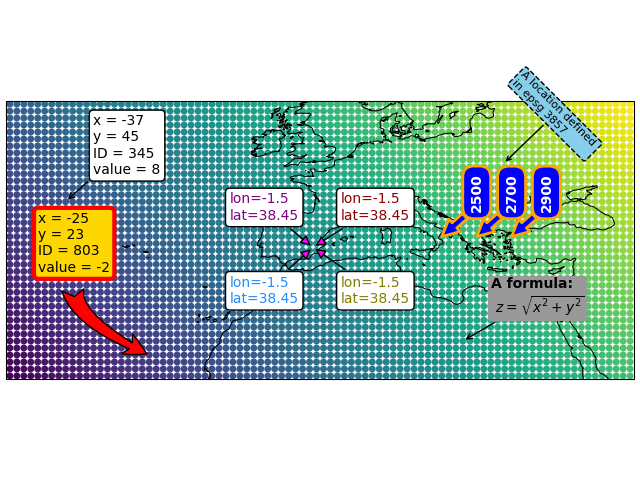### ▭ Rectangular areas

To indicate rectangular areas in any given crs, simply use m.indicate_extent:

 indicate_extent Indicate a rectangular extent in a given crs on the map.
 from eomaps import Maps m = Maps(crs=3035) m.add_feature.preset.coastline(ec="k") # indicate a lon/lat rectangle m.indicate_extent(-20, 35, 40, 50, hatch="//", fc="none", ec="r") # indicate some rectangles in epsg:3035 hatches = ["*", "xxxx", "...."] colors = ["yellow", "r", "darkblue"] for i, h, c in zip(range(3), hatches, colors): pos0 = (2e6 + i*2e6, 7e6, 3.5e6 + i*2e6, 9e6) pos1 = (2e6 + i*2e6, 7e6 + 3e6, 3.5e6 + i*2e6, 9e6 + 3e6) m.indicate_extent(*pos0, crs=3857, hatch=h, lw=0.25, ec=c) m.indicate_extent(*pos1, crs=3857, hatch=h, lw=0.25, ec=c) # indicate a rectangle in Equi7Grid projection try: # (requires equi7grid package) m.indicate_extent(1000000, 1000000, 4800000, 4800000, crs=Maps.CRS.Equi7Grid_projection("EU"), fc="g", alpha=0.5, ec="k") except: pass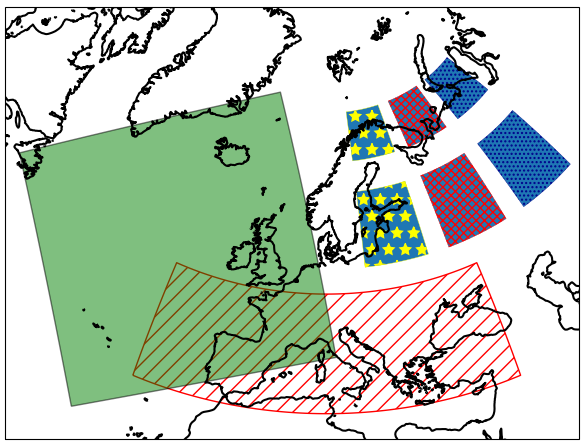## 🌈 Colorbars (with a histogram)

Before adding a colorbar, you must plot the data using m.plot_map().
A colorbar with a colored histogram on top can then be added to the map via m.add_colorbar.

Note

Colorbars are only visible if the layer at which the data was plotted is visible!

m = Maps(layer=0)
...
m.add_colorbar()   # this colorbar is only visible on the layer 0

m2 = m.new_layer("data")
...
m2.add_colorbar()  # this colorbar is only visible on the "data" layer

 Maps.add_colorbar Add a colorbar to an existing figure.
 from eomaps import Maps import numpy as np x, y = np.mgrid[-45:45, 20:60] m = Maps() m.add_feature.preset.coastline() m.set_data(data=x+y, x=x, y=y, crs=4326) m.set_classify_specs(scheme=Maps.CLASSIFIERS.EqualInterval, k=5) m.plot_map() m.add_colorbar(label="what a nice colorbar", histbins="bins")Note

You must plot a dataset first! (e.g. by calling m.plot_map())
The colorbar always represents the dataset that was used in the last call to m.°^^^^plot_map().
If you need multiple colorbars, use an individual Maps object for each dataset! (e.g. via m2  = m.new_layer())

To manually change the position of a previously created colorbar (or the relative size between the colorbar and the histogram), use m.figure.set_colorbar_position().

 set_colorbar_position Set the position (and ratio) of a previously created colorbar with a histogram.

### 🌠 Using the colorbar as a “dynamic shade indicator”

If you use shade_raster or shade_points as plot-shape, the colorbar can be used to indicate the distribution of the shaded pixels within the current field of view by setting dynamic_shade_indicator=True.

 from eomaps import Maps import numpy as np x, y = np.mgrid[-45:45, 20:60] m = Maps() m.add_feature.preset.coastline() m.set_data(data=x+y, x=x, y=y, crs=4326) m.set_shape.shade_raster() m.plot_map() m.add_colorbar(dynamic_shade_indicator=True, histbins=20)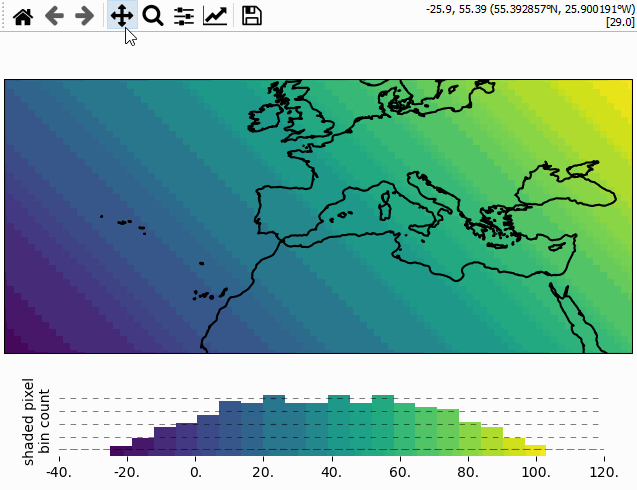## 📏 Scalebars

A scalebar can be added to a map via s = m.add_scalebar():

 Maps.add_scalebar Add a scalebar to the map.
 from eomaps import Maps m = Maps(Maps.CRS.Sinusoidal()) m.add_feature.preset.ocean() s = m.add_scalebar()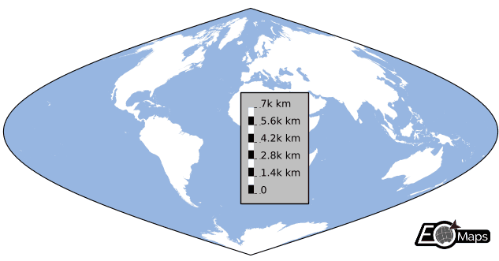Note

The scalebar is a pickable object! Click on it with the LEFT mouse button to drag it around, and use the RIGHT mouse button to make it fixed again.

If the scalebar is picked (indicated by a red border), you can use the following keys for adjusting some of the ScaleBar properties:

• delte: remove the scalebar from the plot

• + and -: rotate the scalebar

• up/down/left/right: increase the size of the frame

• alt + up/down/left/right: decrease the size of the frame

The returned ScaleBar object provides the following useful methods:

 ScaleBar.remove Remove the scalebar from the map ScaleBar.set_position Sset the position of the colorbar ScaleBar.get_position Return the current position (and orientation) of the scalebar (e.g. ScaleBar.set_label_props Set the properties of the labels (and update the plot accordingly) ScaleBar.set_patch_props Set the properties of the frame (and update the plot accordingly) ScaleBar.set_scale_props Set the properties of the scalebar (and update the plot accordingly) ScaleBar.cb_offset_interval Get/set the interval for the text-offset when using the + <+>/<-> keyboard-shortcut to set the offset for the scalebar-labels. ScaleBar.cb_rotate_interval Get/set the interval for the rotation when using the <+> and <-> keys on the keyboard to rotate the scalebar

## 🧭 Compass (or North Arrow)

A compass can be added to the map via m.add_compass():

 Maps.add_compass Add a "compass" or "north-arrow" to the map.
 from eomaps import Maps m = Maps(Maps.CRS.Stereographic()) m.add_feature.preset.ocean() m.add_compass()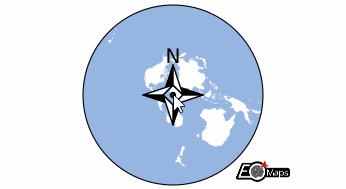The compass object is dynamically updated if you pan/zoom the map, and it can be dragged around on the map with the mouse.

The returned compass object has the following useful methods assigned:

 set_patch Set the style of the background patch. set_scale Set the size of the compass. set_pickable Set if the compass can be picked with the mouse or not. remove Remove the compass from the map.

## 🦜 Utility widgets

Some helpful utility widgets can be added to a map via m.util.<...>

 Maps.util A collection of utility tools that can be added to EOmaps plots

### Layer switching

To simplify switching between layers, there are currently 2 widgets available:

• m.util.layer_selector() : Add a set of clickable buttons to the map that activates the corresponding layers.

• m.util.layer_slider() : Add a slider to the map that iterates through the available layers.

By default the widgets will show all available layers (except the “all” layer).

• To show only a subset of layers, use layers=[...layer names...].

• To exclude certain layers from the widget, use exclude_layers=[...layer-names to exclude...]

 layer_selector Get a button-widget that can be used to select the displayed plot-layer. layer_slider Get a slider-widget that can be used to switch between layers.
 from eomaps import Maps m = Maps(layer="coastline") m.add_feature.preset.coastline() m2 = m.new_layer(layer="ocean") m2.add_feature.preset.ocean() m.util.layer_selector()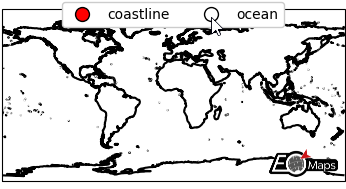## 🔬 Inset-maps - zoom-in on interesting areas

Inset maps that show zoomed-in regions can be created with m.new_inset_map().

m = Maps()                                  # the "parent" Maps-object (e.g. the "big" map)
m2 = m.new_inset_map(xy=(5, 5), radius=10)  # a new Maps-object that represents the inset-map
m2.add_feature.preset.ocean()               # it can be used just like any other Maps-objects!

• An inset-map is defined by it’s center-position and a radius

• The used boundary-shape can be one of:

• “ellipses” (e.g. projected ellipses with a radius defined in a given crs)

• “rectangles” (e.g. projected rectangles with a radius defined in a given crs)

• “geod_circles” (e.g. geodesic circles with a radius defined in meters)

For convenience, inset-map objects have the following special methods defined:

• m.set_inset_position(): Set the size and (center) position of the inset-map relative to the figure size.

• m.indicate_inset_extent(): Indicate the extent of the inset-map on arbitrary Maps-objects.

Checkout the associated example on how to use inset-maps: 🔬 Inset-maps - get a zoomed-in view on selected areas

 m = Maps(Maps.CRS.PlateCarree(central_longitude=-60)) m.add_feature.preset.ocean() m2 = m.new_inset_map(xy=(5, 45), radius=10, plot_position=(.3, .5), plot_size=.7, edgecolor="r", linewidth=4, indicate_extent=dict(fc=(1,0,0,.5), ec="r", lw=1) ) m2.add_feature.preset.coastline() m2.add_feature.preset.countries() m2.add_feature.preset.ocean() m2.add_feature.cultural_10m.urban_areas(fc="r") m2.add_feature.physical_10m.rivers_europe(ec="b", lw=0.25) m2.add_feature.physical_10m.lakes_europe(fc="b")new_inset_map Create a new (empty) inset-map that shows a zoomed-in view on a given extent.

## 📦 Reading data (NetCDF, GeoTIFF, CSV…)

EOmaps provides some basic capabilities to read and plot directly from commonly used file-types.

By default, the Maps.from_file and m.new_layer_from_file functions try to plot the data with shade_raster (if it fails it will fallback to shade_points and finally to ellipses).

Note

The readers are intended for well-structured datasets! If they fail, simply read and extract the data manually and then set the data as usual via m.set_data(...).

Under the hood, EOmaps uses the following libraries to read data:

• GeoTIFF (rioxarray + xarray.open_dataset)

• NetCDF (xarray.open_dataset)

• CSV (pandas.read_csv)

### Read relevant data from a file

m.read_file.<filetype>(...) can be used to read all relevant data (e.g. values, coordinates & crs) from a file.

m = Maps()
"the filepath",
coords=("longitude", "latitude"),
data_crs=4326,
isel=dict(time=123)
)
m.set_data(**data)
...
m.plot_map()

 read_file.GeoTIFF Read all relevant information necessary to add a GeoTIFF to the map. read_file.NetCDF Read all relevant information necessary to add a NetCDF to the map. read_file.CSV Read all relevant information necessary to add a CSV-file to the map.

### Initialize Maps-objects from a file

Maps.from_file.<filetype>(...) can be used to directly initialize a Maps object from a file. (This is particularly useful if you have a well-defined file-structure that you need to access regularly)

m = Maps.from_file.GeoTIFF(
"the filepath",
classify_specs=dict(Maps.CLASSFIERS.Quantiles, k=10),
cmap="RdBu"
)
m.cb.pick.attach.annotate()

 from_file.GeoTIFF Convenience function to initialize a new Maps-object from a GeoTIFF file. from_file.NetCDF Convenience function to initialize a new Maps-object from a NetCDF file. from_file.CSV Convenience function to initialize a new Maps-object from a CSV file.

### Add new layers to existing Maps-objects from a file

Similar to Maps.from_file, a new layer based on a file can be added to an existing Maps object via Maps.new_layer_from_file.<filetype>(...).

m = Maps8()

m2 = m.new_layer_from_file(
"the filepath",
coords=("longitude", "latitude"),
data_crs=4326,
isel=dict(time=123),
classify_specs=dict(Maps.CLASSFIERS.Quantiles, k=10),
cmap="RdBu"
)

 new_layer_from_file.GeoTIFF Convenience function to initialize a new Maps-object from a GeoTIFF file. new_layer_from_file.NetCDF Convenience function to initialize a new Maps-object from a NetCDF file. new_layer_from_file.CSV Convenience function to initialize a new Maps-object from a CSV file.

## 🔸 Miscellaneous

some additional functions and properties that might come in handy:

 Maps.join_limits Join the x- and y- limits of the axes (e.g. Maps.get_crs get the pyproj CRS instance of a given crs specification Maps.indicate_masked_points Add circles to the map that indicate masked points. Maps.BM The Blit-Manager used to dynamically update the plots Maps.parent The parent-object to which this Maps-object is connected to. Maps.crs_plot The crs used for plotting. Maps.add_colorbar Add a colorbar to an existing figure. Maps.show Make the layer of this Maps-object visible. Maps.show_layer Display the selected layer on the map.